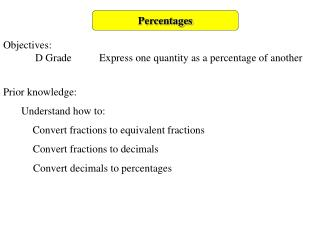DownloadDownload PresentationPercentages

# Percentages

Download Presentation## Percentages

- - - - - - - - - - - - - - - - - - - - - - - - - - - E N D - - - - - - - - - - - - - - - - - - - - - - - - - - -
##### Presentation Transcript

1. Percentages Objectives: D Grade Express one quantity as a percentage of another Prior knowledge: Understand how to: Convert fractions to equivalent fractions Convert fractions to decimals Convert decimals to percentages

2. Percentages What is a percentage? percent per cent out of hundred So we can think of percentages also as fractions with a denominator of 100: This helps us to understand that finding a percentage of a quantity is the same as finding a fraction of a quantity e.g. 65 percent 65 65 per 0 00 0 cent

3. Percentages Expressing one quantity as a percentage of another Compare one quantity with the other. e.g. compare 20 with 25 20 Write: compare with 25 Write as an equivalent fraction with the denomination 100 20 80 ≡ 100 25 This is now 80% because 80 is the same as 80% 100

4. Percentages Sometimes you might need to have an intermediate equivalent fraction e.g. compare 4 with 40 4 Write: compare with 40 Simplify as an equivalent fraction ≡ Now write as an equivalent fraction with the denominator of 100 4 1 10 1 10 100 40 10 ≡ This is now 10% because 10 is the same as 10% 100

5. Percentages Now do these: 1. Write 4 as a percentage of 20 2. Write 50 as a percentage of 200 3. Write 14 as a percentage of 28 4. Write 4 as a percentage of 25 5. Write 18 as a percentage of 45 ≡ ≡ 2 3 10 4 16 21 50 25 4 40 20 18 5 4 100 100 100 100 100 25 28 45 200 20 ≡ ≡ ≡ ≡ ≡

6. Percentages Harder percentages of quantities can be found using a calculator. 24 can be read as ÷ compare with 34 Convert from a fraction to a decimal: Using a calculator: 24 ÷ 34 = 0.7059 Convert from a decimal to a percentage: 0.7059 × 100 = 70.59 70.59 %

7. Percentages Now do these: 1. Write 3 as a percentage of 72 2. Write 42 as a percentage of 76 3. Write 45 as a percentage of 135 4. Write 37 as a percentage of 52 5. Write 92 as a percentage of 56 3 ÷ 72 = 0.04167 45 ÷ 135 = 0.3333 37 ÷ 52 = 0.7115 42 ÷ 76 = 0.5526 92 ÷ 56 = 1.6429 0.04167 × 100 = 4.167 % 0.7115 × 100 = 71.15 % 0.3333 × 100 = 33.33 % 0.5526 × 100 = 55.26 % 1.6429 × 100 = 164.29 %